# CS 61 Programming Assignment 05

## Objective

The purpose of this final programming assignment is to test the limits of your LC3 skill by giving you a challenging implementation problem straight out of the textbook.

## High Level Description

There is a server somewhere, called the Busyness Server. This server tracks whether 16 different machines connected to it are busy (0) or free (1).

You will write a menu-driven system that allows the user to query the availability of the 16 machines in various combinations.

## Before You Start Coding

This assignment is based on a variation of Question 9.9 (p. 242 of the textbook) – which will require that you first work through the following: ● Example 2.11 (p. 37)

• Question 2.36 (p. 46)
• Question 5.6 (p. 146)

## Example 2.11

Suppose we have eight machines that we want to monitor with respect to their availability. We can keep track of them with an 8-bit BUSYNESS vector, where a bit is 1 if the unit is free and 0 if the unit is busy. The bits are labeled, from right to left, from 0 to 7.

The BUSYNESS bit vector 11000010 corresponds to the situation where only units 7, 6, and 1 are free, and therefore available for work assignment.

Suppose work is assigned to unit 7. We update our BUSYNESS bit vector by performing the logical AND, where our two sources are the current bit vector 11000010 and the bit mark 01111111. The purpose of the bit mask is to clear bit 7 of the BUSYNESS bit vector. The result is the bit vector 01000010.

Recall that we encountered the concept of bit mask in Example 2.7. Recall that a bit mask enables one to interact some bits of a binary pattern while ignoring the rest. In this case, the bit mask clears bit 7 and leaves unchanged (ignores) bits 6 through 0.

Suppose unit 5 finishes its task and becomes idle. We can update the BUSYNESS bit vector by performing the logical OR of it with the bit mask 00100000. The result is 01100010.

## Question 2.36

Refer to Example 2.11 for the following questions.

1. What mask value and what operation would one use to indicate that machine 2 is busy?
2. What mask value and what operation would one use to indicate that machines 2 and 6 are no longer busy? (Note: This can be done with only one operation)
3. What mask value and what operation would one use to indicate that all machines are busy? D. What mask value and what operation would one use to indicate that all machines are idle (not busy)?
4. Develop a procedure to isolate the status bit of machine 2 as the sign bit. For example, if the

BUSYNESS pattern is 01011100, then the output of this procedure is 10000000. If the BUSYNESS pattern is 01110011, then the output is 00000000. In general, if the BUSYNESS pattern is:

 b7 b6 b5 b4 b3 b2 b1 b0

the output is

 b2 0 0 0 0 0 0 0

Hint: What happens when you ADD a bit pattern to itself?

## Question 5.6

Recall the machine busy example from Section 2.7.1. Assuming the BUSYNESS bit vector is stored in R2, we can use the LC3 instruction 0101 001 010 1 0001 (AND R3, R2, #1) to determine whether machine 0 is busy or not. If the result of this instruction is 0, then machine 0 is busy.

1. Write an LC3 instruction that determines whether machine 2 is busy.
2. Write an LC3 instruction that determines whether both machines 2 and 3 are busy.
3. Write an LC3 instruction that indicates whether none of the machines are busy.
4. Can you write an LC3 instruction that determines whether machine 6 is busy? Is there a problem here?

The variation of Question 9.9 that you will implement in this assignment:

1. Check if all machines are busy; return 1 if all are busy, 0 otherwise.
2. Check if all machines are free; return 1 if all are free, 0 otherwise.
3. Check how many machines are busy; return the number of busy machines.
4. Check how many machines are free; return the number of free machines.
5. Check the status of a specific machine whose number is passed as an argument in R1; return 1 if that machine is free, 0 if it is busy.
6. Return the number of the first (lowest numbered) machine that is free.

If no machine is free, return -1

The assignment can be broken down into the following tasks:

1. Your main code block should call a MENU subroutine, which prints out a fancy looking menu with numerical options, allows the user to input a choice, and returns in R1 the choice (i.e. 1, 2, 3, 4, 5, 6, 7) that the user made (if the user inputs a non-existent option, output error message and the menu will simply repeat until a valid entry is obtained).

Here is the menu that you will be using for the assignment. It is given to you in the template at memory address x6000

***********************

* The Busyness Server *

***********************

1. Check to see whether all machines are busy
2. Check to see whether all machines are free
3. Report the number of busy machines
4. Report the number of free machines
5. Report the status of machine n
6. Report the number of the first available machine
7. Quit
1. Write the following subroutines:
2. ALL_MACHINES_BUSY
3. ALL_MACHINES_FREE
4. NUM_BUSY_MACHINES
5. NUM_FREE_MACHINES
6. MACHINE_STATUS
7. FIRST_FREE

The following headers have been provided to you in the given template.

; Inputs: None

; Postcondition: The subroutine has printed out a menu with numerical ; options, captured the user selection, and returned it.

; Return Value (R1): The option selected: #1, #2, #3, #4, #5, #6 or #7

; no other return value is valid

; Subroutine: ALL_MACHINES_BUSY

; Inputs: None

; Postcondition: The subroutine has returned a value

; indicating whether all machines are busy

; Return value (R2): 1 if all machines are busy, 0 otherwise

; Subroutine: ALL_MACHINES_FREE

; Inputs: None

; Postcondition: The subroutine has returned a value

; indicating whether all machines are free

; Return value (R2): 1 if all machines are free, 0 otherwise

; Subroutine: NUM_BUSY_MACHINES

; Inputs: None

; Postcondition: The subroutine has returned the number of busy machines. ; Return Value (R2): The number of machines that are busy

; Subroutine: NUM_FREE_MACHINES

; Inputs: None

; Postcondition: The subroutine has returned the number of free machines ; Return Value (R2): The number of machines that are free

; Subroutine: MACHINE_STATUS

; Input (R1): Which machine to check

; Postcondition: The subroutine has returned a value

; indicating whether or not machine (R1) is busy

; Return Value (R2): 0 if machine (R1) is busy, 1 if it is free

; Subroutine: FIRST_FREE

; Inputs: None

; Postcondition: The subroutine has returned a value

; indicating the lowest numbered free machine

; Return Value (R2): the number of the free machine

### Expected/ Sample output

Output

• See provided template and examples below.

## Examples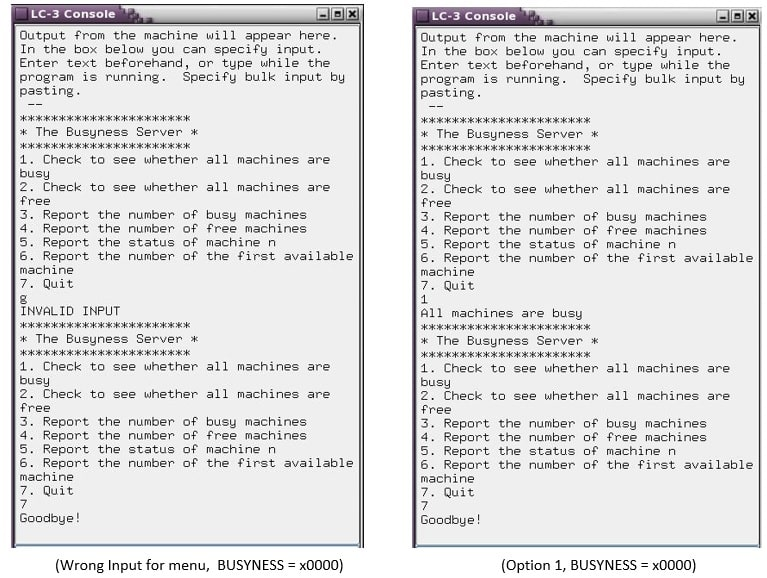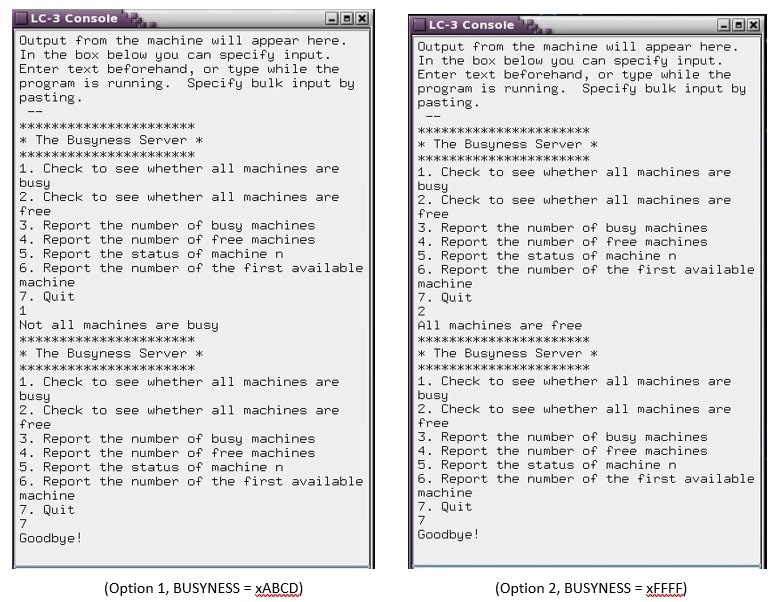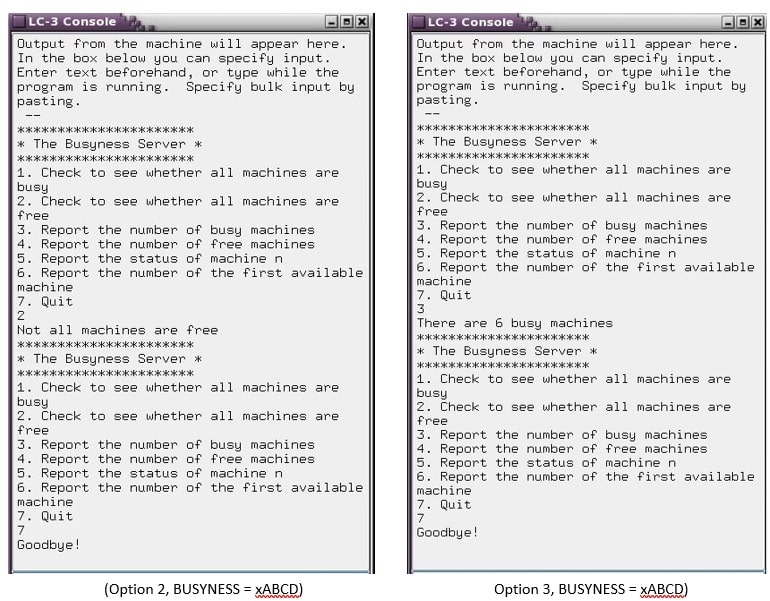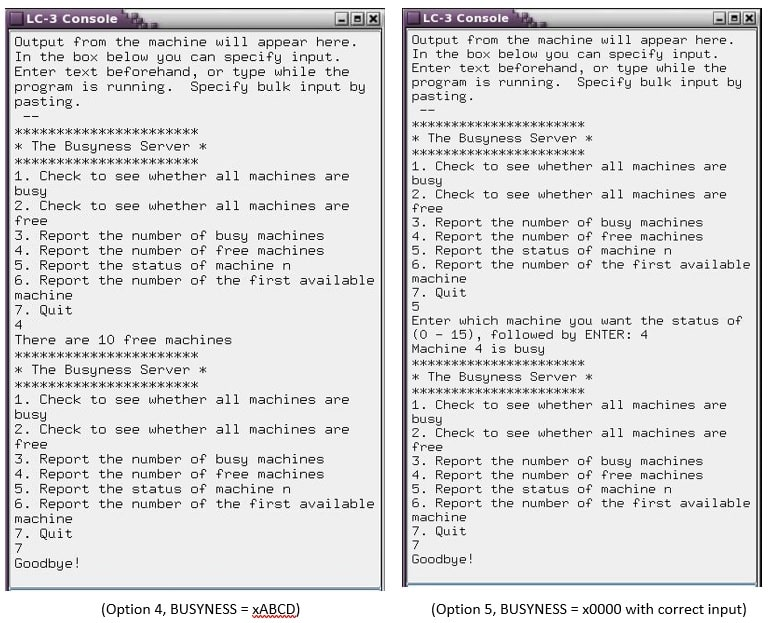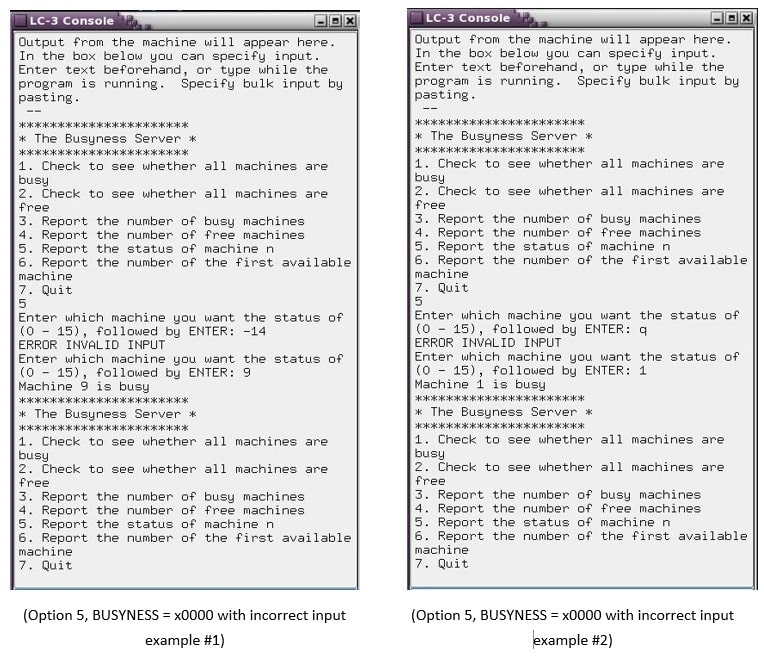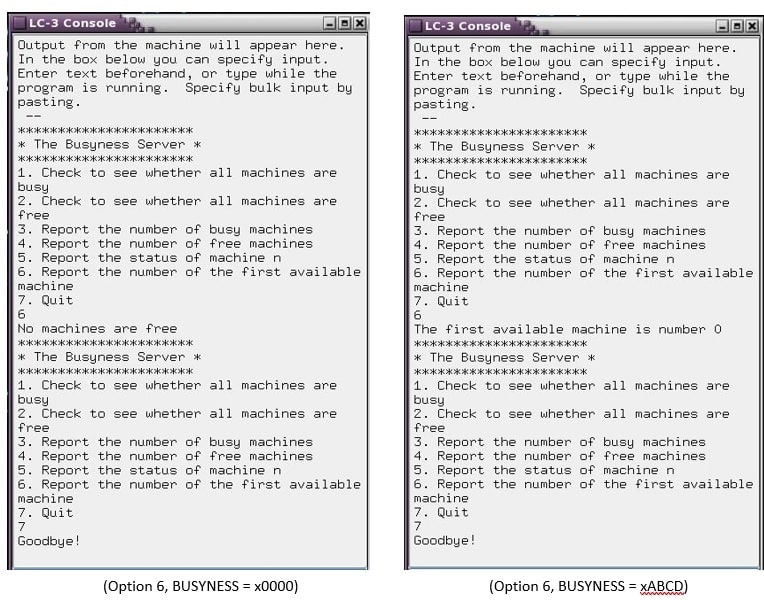Notes:

• As always, you must echo the digits as they are input (no "ghost writing").
• When an invalid input is detected: Output the error message and reprompt the user for input
• NO leading zeros on output numbers (you must still echo any leading zeros the user inputs)
• When outputting positive response there is no sign ( though a '+' sign may be used on input)

## REMEMBER: all outputs must be newline terminated

Your code will obviously be tested with a range of different values: Make sure you test your code likewise!

Uhhelp?

This is actually not a difficult assignment – but we’ll give you some hints anyway :)

Really, Really Important

#### 1 means free; 0 means busy

• Store the BUSYNESS vector at the memory address given to you in the template. ○ The grader will test different values using this address Hint 01

Remember the algorithm for printing a number out in binary. Remember how to examine each individual bit of a 16-bit value (i.e. any number in a register).

Hint 02

Steal the headers we wrote and use them to help guide your step-by-step completion of the assignment (yay - endorsed micro-plagiarism?)

Hint 03 – sub A (MENU)

The MENU subroutine gets a single character from the user. You do NOT have to press enter for the character to be accepted. The moment the user enters a character, the subroutine will try and validate the input, if valid will return the option value. If not then an error message should be outputted and start the process from the beginning.

Hint 04 – subs B & C

Notice that if a machine is free, then it is NOT busy. If a machine is busy, then it is NOT free - emphasis on the keyword “NOT” :)

Hint 05 – subs D & E

If N = the total number of machines (16), and X = the total number of free machines, and Y = the total number of busy machines,

then note that X = 16 – Y and Y = 16 – X

Hint 06 – subs F & GFrom the specs:

; Postcondition: The subroutine has returned a value

; indicating the lowest numbered free machine

In other words, The FIRST machine is machine 0, the LAST machine is machine 15.

Machine 15 is MSB and Machine 0 is LSB

Machine IDs:

Subroutine F (MACHINE STATUS):

Examples of valid input:

• 12
• 0
• 0003

Examples of invalid input:

• -12
• 16
• +2g
• g

Note: The only acceptable values are between 0 and 15 .

Hint 07

Do not forget to follow all of the steps of the subroutine construction process.

Hint 08

Did we mention that 1 means free; 0 means busy ?

Hint 09

To implement your menu-driven system, implement an infinite loop that works like this:

while( true )

{`{`}

if (choice == 1)

R2 = all_machines_busy() ; call ALL_MACHINES_BUSY subroutine if (R2 == 1)

Print “All machines are busy”[ else

Print “Not all machines are busy”

else if (choice == 2)

[similar]

else if (choice == 3)

R2 = num_busy_machines() ; call NUM_BUSY_MACHINES subroutine

Print “There are “, (R2), “ machines busy”

else if (choice == 4)

[similar]

else if (choice == 5)

Print “Which machine do you want the status of (0 - 15)?”

; NOTE: you may want to build a “helper” subroutine

; to obtain validated input here

R1 = <validated user entry from #0 to #15>

R2 = machine_status(R1) ; call MACHINE_STATUS and pass R1 if (R2 == 1)

Print “Machine “, (R1), “ is free” else

Print “Machine “, (R1), “ is busy”

else if (choice == 6)

R2 = first_machine_free() ; call FIRST_Free subroutine

if (R2 == <come up with a sentinel value> )

Print “No machines are free”

else

Print “The first available machine is number”, (R2)

else if (choice == 7)

Print “Goodbye!”

{`HALT }`}

Hint 10

Use the template provided. It’s given for a reason.

Submission Instructions

Submit to GitHub for testing, feedback and grading.